# HSSlive: Plus One & Plus Two Notes & Solutions for Kerala State Board

## BSEB Class 9 Maths Chapter 15 Probability Ex 15.1 Textbook Solutions PDF: Download Bihar Board STD 9th Maths Chapter 15 Probability Ex 15.1 Book AnswersBSEB Class 9 Maths Chapter 15 Probability Ex 15.1 Textbook Solutions PDF: Download Bihar Board STD 9th Maths Chapter 15 Probability Ex 15.1 Book Answers

BSEB Class 9th Maths Chapter 15 Probability Ex 15.1 Textbooks Solutions and answers for students are now available in pdf format. Bihar Board Class 9th Maths Chapter 15 Probability Ex 15.1 Book answers and solutions are one of the most important study materials for any student. The Bihar Board Class 9th Maths Chapter 15 Probability Ex 15.1 books are published by the Bihar Board Publishers. These Bihar Board Class 9th Maths Chapter 15 Probability Ex 15.1 textbooks are prepared by a group of expert faculty members. Students can download these BSEB STD 9th Maths Chapter 15 Probability Ex 15.1 book solutions pdf online from this page.

## Bihar Board Class 9th Maths Chapter 15 Probability Ex 15.1 Books Solutions

 Board BSEB Materials Textbook Solutions/Guide Format DOC/PDF Class 9th Subject Maths Chapter 15 Probability Ex 15.1 Chapters All Provider Hsslive

## How to download Bihar Board Class 9th Maths Chapter 15 Probability Ex 15.1 Textbook Solutions Answers PDF Online?

2. Click on the Bihar Board Class 9th Maths Chapter 15 Probability Ex 15.1 Answers.
3. Look for your Bihar Board STD 9th Maths Chapter 15 Probability Ex 15.1 Textbooks PDF.
4. Now download or read the Bihar Board Class 9th Maths Chapter 15 Probability Ex 15.1 Textbook Solutions for PDF Free.

Find below the list of all BSEB Class 9th Maths Chapter 15 Probability Ex 15.1 Textbook Solutions for PDF’s for you to download and prepare for the upcoming exams:

## BSEB Bihar Board Class 9th Maths Solutions Chapter 15 Probability Ex 15.1

Question 1.
In a cricket match, a batswoman hits a boundary 6 times out of 30 balls she plays. Find the probability that she did not hit a boundary.
Solution:
Since in a cricket match, a batswoman hits a boundary 6 times out of 30 balls she plays i.e., she missed the boundary 30 – 6 = 24 times out of 30 balls.
∴ The probability that a batswoman does not hit a boundary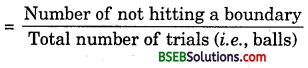2430 i.e., 45

Question 2.
1500 families with 2 children were selected randomly, and the following were recorded: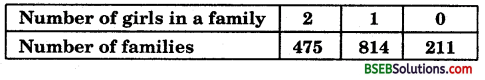Compute the probability of a family, chosen at random, having
(i) 2 girls
(ii) 1 girl
(iii) No girl
Also check whether the sum of these probabilities is 1.
Solution:
Let E0, E1 and E2 be the event of getting no girl, 1 girl and 2 girls.
(i) P(E2) = Probability of a family having 2 girls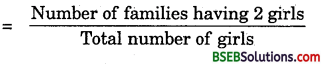4751500 = 1960

(ii) P(E1) = Probability of a family having 1 girl8141500 = 407750

(iii) P(E0) = Probability of a family having no girls2111500
∴ sum of probabilities = P(E0) + P(E1) + P(E2)
= 2111500 + 407750 + 1960
= 211+814+4751500 = 15001500
= 1

Question 3.
Refer to Example 5, section 14.4, Chapter 14. Find the probability that a student of the class was born in August.
Solution:
Clearly from the histograph six students were born in the month of August out of 40 students of a particular section of class IX.
Probability that a student of the class was born in August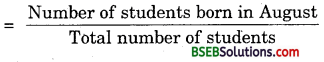= 640
= 320

Question 4.
Three coins are tossed simultaneously 200 times with the following frequencies of different outcomes: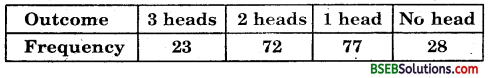If the three coins are simultaneously tossed again, compute the probability of 2 heads coming up.
Solution:
Since three coins are tossed 200 times, so the total number of trials is 200.Total no. of trials
= 72200
= 925

Question 5.
An organisation selected 2400 families at random and surveyed them to determine a relationship between income level and the number of vehicles in a family.
The information gathered is listed in the table below:Suppose a family is chosen. Find the probability that the family chosen is
(i) earning Rs 10000 – 13000 per month and owning exactly 2 vehicles.
(ii) earning Rs 16000 or more per month and owning exactly 1 vehicle.
(iii) earning less than Rs 7000 per month and does not own any vehicle.
(iv) earning Rs 13000 – 16000 per month and owning more than 2 vehicles.
(v) owning not more than 1 vehicle.
Solution:
The total number of families = 2400
(i) Number of families earning Rs 10000 – 13000 per month and owning exactly 2 vehicles = 29.
∴ P (Families earning Rs 10000 – 13000 per month and owning exactly 2 vehicles)
= 292400

(ii) Number of families earning Rs 16000 or more per month and owning exactly 1 vehicle = 579.
∴ P (Families earning Rs 16000 or more per month and owning exactly 1 vehicle)
= 5792400

(iii) Number of families earning less than Rs 7000 per . month and does not own any vehicle =10.
∴ P (Families earning.less than Rs 7000 per month and does not own aify vehicle)
= 1002400
= 1240

(iv) Number of families earning Rs 13000 – 16000 per month and owning more than 2 vehicles = 25
∴ P (Families earning Rs 13000 – 16000 per month and owing more than 2 vehicles) = 252400 = 196

(v) Number of families owning not more than 1 vehicle = Families having no vehicle + Families having 1 vehicle
= (10 + 0 + 1 + 2 + 1) + (160 + 305 + 535 + 469 + 579)
= 14 + 2148 = 2162
P (Families owning not more than 1 vehicle)
= 21622400 = 10311200

Question 6.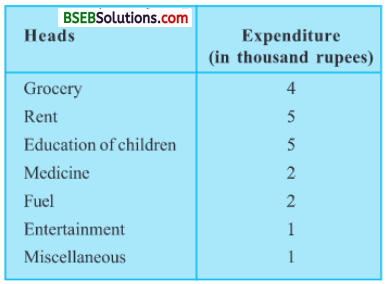(i) Find the probability that a student obtained less than 20% in the mathematics test.
(ii) Find the probability that a student obtained marks 60 or above.
Solution:
Total number of students in mathematics is 90.
(i) Clearly, from the given table, the number of student who obtained less than 20% marks in the mathematics test = 7.
P (a student obtaining less than 20% marks) = 790

(ii) Clearly, from the given table, number of students who obtained marks 60 or above
= (students in 60-70) + (students above 70)
= 15 + 8 = 23
∴ P (a student obtaining marks 60 and above) = 2390

Question 7.
To know the opinion of the students about the subject statistics, a survey of 200 students was conducted. The data is recorded in the following table.

 Opinion Number of students like 135 dislike 65

Find the probability that a student chosen at random
(i) likes statistics, (ii) does not like it.
Solution:
The total number of students = 200
(i) P (a student likes statistics)= 135200 = 2740

(ii) P (a student does not like statistics)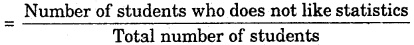= 65200 = 1340

Question 8.What is the empirical probability that an engineer lives:
(i) less than 7 km from her place of work?
(ii) more than or equal to 7 km from her place of work?
(iii) within 12 km from her place of work?
Solution:
Total number of engineers = 40
(i) Number of engineers living less than 7 km from their place of work = 9
∴ P (an engineer lives less than 7 km from her place of work)
= 940

(ii) Number of engineers living more than or equal to 7 km from her place of work
= 31
∴ P (an engineer lives less than or equal to 7 km from her place of work)
= 3140

(iii) Number of engineer lives within 12 km from her place of work = 0
∴ P (an engineer lives with 12 km from her place of work) = 040 = 0

Question 9.
Activity : Note the frequency of two-wheelers, three-wheelers and four-wheelers going past during a time interval, in front of your school gate. Find the probability that any one vehicle out of the total vehicles you have observed is a two-wheeler.
Solution:
Activity problem : Collect the data and find the desired probability.

Question 10.
Activity : Ask all the students in your class to write a 3-digit number. Choose any student from the room at random. What is the probability that the number written by her/him is divisible by 3 ? Remember that a number is divisible by 3, if the sum of its digits is divisible by 3.
Solution:
Activity problem : Do as directed and find the desired probability.

Question 11.
Eleven bags of wheat flour, each marked 5 kg, actually contained the following weights of flour (in kg) :
4.97, 5.05, 5.08, 5.03, 5.00, 5.06, 5.08, 4.98, 5.04, 5.07, 5.00
Find the probability that any of these bags chosen at random contains more than 5 kg of flour.
Solution:
Total number of wheat bags = 11
Number of bags having more than 5 kg = 7
∴ P (a bag contains more than 5 kg) = 711

Question 12.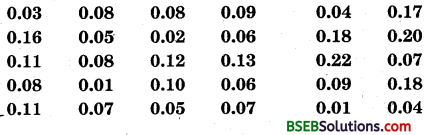you were asked to prepare a frequency distribution table, regarding the concentration of sulphur dioxide in the air in parts per million of a certain city for 30 days. Using this table, find the probability of the concentration of sulphur dioxide in the interval 0.12 – 0.16 on any of these days.
Solution:
Total number of days = 30
Concentration of sulphur dioxide in 0.12 – 0.16 on any day = 2
∴ Required probability = 230 = 115

Question 13.
A, B, O, O, AR, O, A, O, B, A, O, B, A, O, O, A, AB, O, A, A, O, O, AB, B, A, O, B, A, B, O.
you were asked to prepare a frequency distribution table regarding the blood groups of 30 students of a class. Use this table to determine the probability that a student of this class, selected at random, has blood group AB.
Solution:
Total number of students = 30
Number of students having blood group AB = 3
∴ Required probability = 330 = 110

## Bihar Board Class 9th Maths Chapter 15 Probability Ex 15.1 Textbooks for Exam Preparations

Bihar Board Class 9th Maths Chapter 15 Probability Ex 15.1 Textbook Solutions can be of great help in your Bihar Board Class 9th Maths Chapter 15 Probability Ex 15.1 exam preparation. The BSEB STD 9th Maths Chapter 15 Probability Ex 15.1 Textbooks study material, used with the English medium textbooks, can help you complete the entire Class 9th Maths Chapter 15 Probability Ex 15.1 Books State Board syllabus with maximum efficiency.

## FAQs Regarding Bihar Board Class 9th Maths Chapter 15 Probability Ex 15.1 Textbook Solutions

#### Can we get a Bihar Board Book PDF for all Classes?

Yes you can get Bihar Board Text Book PDF for all classes using the links provided in the above article.

## Important Terms

Bihar Board Class 9th Maths Chapter 15 Probability Ex 15.1, BSEB Class 9th Maths Chapter 15 Probability Ex 15.1 Textbooks, Bihar Board Class 9th Maths Chapter 15 Probability Ex 15.1, Bihar Board Class 9th Maths Chapter 15 Probability Ex 15.1 Textbook solutions, BSEB Class 9th Maths Chapter 15 Probability Ex 15.1 Textbooks Solutions, Bihar Board STD 9th Maths Chapter 15 Probability Ex 15.1, BSEB STD 9th Maths Chapter 15 Probability Ex 15.1 Textbooks, Bihar Board STD 9th Maths Chapter 15 Probability Ex 15.1, Bihar Board STD 9th Maths Chapter 15 Probability Ex 15.1 Textbook solutions, BSEB STD 9th Maths Chapter 15 Probability Ex 15.1 Textbooks Solutions,
Share: